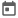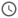# Verilog Event Semantics##### 21 Sep 2020##### 4 mins

Digital devices have input and output as signals, and we know signals are anything that changes their value with time. Thus Verilog is a time-dependent simulator that is based on the C language. A Verilog simulation runs infinitely in a loop unless stopped, simulating the output for every input.

### Program Flow

As seen earlier, Verilog is a time-dependent simulator. Thus, we define the simulator’s time step to sample the input and calculate the output for every code we write in Verilog. This time is nothing but the precision in which the simulation will have.

For example, let us suppose we have to design a sequential circuit whose clock is 5ns. Thus, to model it correctly, we need to have at least 5ns as the time step.

Also, this time step should not be confused with the real-time interval, as the program will also take time to execute codes and thus, it may be possible that it would more time to advance to the next step.

For example, let us suppose we have a time step of 1ns. Thus, Verilog will sample all the values at an interval of 1 ns and give the output. However, it is not guaranteed that the simulation time interval of 1ns will match the actual time interval of 1ns.

The reason for this is that the Verilog is itself running on a processor, and Verilog would require some time to carry out the calculations in each time step, and this calculation time may be greater than 1ns. Once the calculation is done for a particular time step, the simulation goes to the next step, and the cycle continues until the simulation ends.
In this graph, we can see that different calculation points have different calculation times. Thus the simulation time step is a hypothetical time step in which the hardware is simulated.

### Event Semantics

In Verilog, each time step is divided into 4 different regions in which various statements are executed to simulate the hardware accurately. Thus, for every time step, the calculations or simulations are done in 4 steps, known as event semantics. These regions are

1. Active region
2. In-active region
3. NBA or Non-Blocking assignment region
4. Postponed region.

Understanding the concept of event semantics is vital as it helps to avoid race round conditions while designing.

When the simulation moves to a new timestep, first, the active region is executed. In this region, all the code except few system functions and NBAs gets executed, and the order of execution is not defined. Then In-active region is executed. In this region, #0 delay statements are executed. After this NBA region is executed in which LHS of NBA statements are assigned. NBA region marks the end of all calculations. The postponed region which comes next is used to monitor the signals. Some of the system function like `\$monitor`, `\$strobe` uses this region to display a message in the command line.